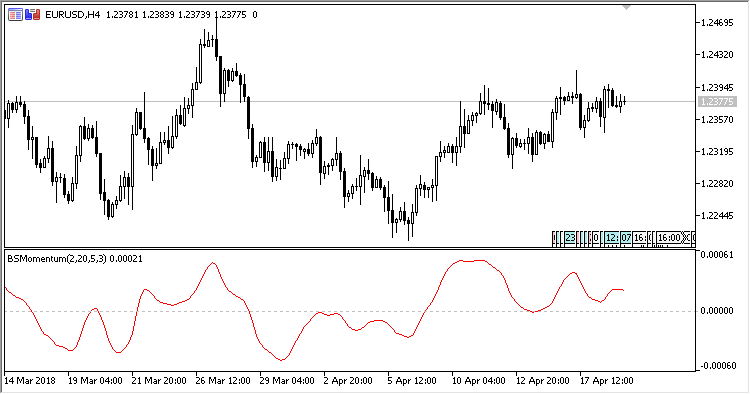# Blau_SM – indicator for MetaTrader 5

• A+
category：MT5 INDICATORS

The William Blau Stochastic Momentum indicator shows the William Blau stochastic momentum.

It has five input parameters:

• Period - calculation period;
• First smoothing period - the initial smoothin period;
• Second smoothing period - the secondary smoothing period;
• Third smoothing period - the resulting smoothing period;
• Applied price - price used for calculations.

Calculations:

`Blau_SM = EMA(EMA(EMA(HH, Smooth period 1), Smooth period 2), Smooth period 3)`

where:

```HH[i] = Price[i]-(Max+Min)/2,
Max, Min are the highest and the lowest price within the range from (i-Period+1) to (i)
```